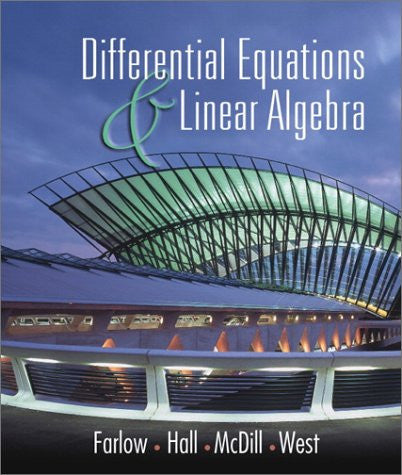# Differential Equations and Linear Algebra

• \$72.57
• Save \$9.50
• Binding: Hardcover
• Author: Jerry Farlow;James E. Hall;Jeanie McDill;Beverly H. West;Jean Marie McDill
• Publish Date: 2001-09-18

Attention : For textbook, access codes and supplements are not guaranteed with used items.

For Differential Equation and Linear Algebra courses at a sophomore level.Using a unique, student-friendly approach to teaching differential equations, this text encourages students to think both quantitatively and qualitatively when approaching differential equations. Before finding the analytical solution of a differential equation, the text presents the qualitative aspects of the problem--the directional field, the bounded solutions, their range, the presence of constant solutions and so on--to help students use linear algebra to think about the physical systems being modeled. In addition, this text features a fluid integration of linear algebra therefore emphasizing the inter-relatedness of differential equations and linear algebra.